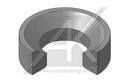0 0
Rubber products

# PF-007(0)
\$0.00
pcs.
Nomenclatures
Article Material Weight (g) Standard Description
PF-007-001 EPDM 70 - - D=35 d=24,5 d1=16 H=11,5
PF-007-003 EPDM 70 - - D=36,9 d=31,6 d1=17,7 H=8,1
PF-007-004 EPDM 70 - - D=32 d=16,9 d1=? H=13,5
PF-007-002 EPDM 70 - - D=34 d=27,8 d1=16,5 H=8
PF-007-000 EPDM 70 - - D=39,8 d1=18 H=9,8
Description
Nomenclatures
Article Material Weight (g) Standard Description
PF-007-001 EPDM 70 - - D=35 d=24,5 d1=16 H=11,5
PF-007-003 EPDM 70 - - D=36,9 d=31,6 d1=17,7 H=8,1
PF-007-004 EPDM 70 - - D=32 d=16,9 d1=? H=13,5
PF-007-002 EPDM 70 - - D=34 d=27,8 d1=16,5 H=8
PF-007-000 EPDM 70 - - D=39,8 d1=18 H=9,8